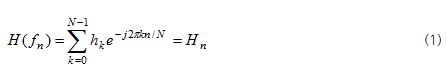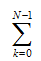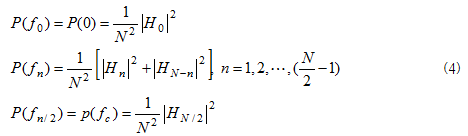In general, data are time series data changing over time. Such data of time domain are useful in observing the change pattern of data over time, but analysis only at time domain is not sufficient when finding important information and analyzing it. Transformation of time series signals into frequency domain will enable you to identify frequency components of signals. So, you can conduct various analysis. At this time, the important problem is how such data of time domain can be transformed into frequency domain. This process is the very Fast Fourier Transform (FFT) described earlier. With regard to FFT, please refer to Frequency Filter.

Power Spectrum is a method widely used in various fields, such as bio signals, video signals, audio signals, and communication signals. This method has a wide variety of application fields and so, there are many terms describing this method, including Power Spectral Density (PSD), Periodogram, and Spectrum Normalization. Here, we use the term of Power Spectrum. Depending on expression methods,

Power Spectrum can be classified into one-side Power Spectrum and two-side Power Spectrum. One-side Power Spectrum shows frequency domains of 0 and positive domain, while two-side Power Spectrum shows all frequency domains of negative, 0, and positive domains. One-side Power Spectrum generally used for data analysis will be explained here. Power Spectrum is obtained using Fourier Transform. Discrete Fourier Transform (DFT) for discrete digital signals can be defined as follow;By Inverse Fast Fourier Transform (IFFT), this formula can be expressed as follow;From the above formula (2), the following formula (3) is derived by obtaining absolute values of both sides, squaring them, applyingand summing all. As you can see in the below formula (3), the sum of square of original signals is identical to the square of signals gone through Fourier Transform. The sum of square of original signals or sum of Fourier Transform is called as Total Power. In other words, total power of signals is all the same at time and frequency spaces, which is called as Parseval theorem.One-side Power Spectrum satisfying this theorem can be defined as follow;